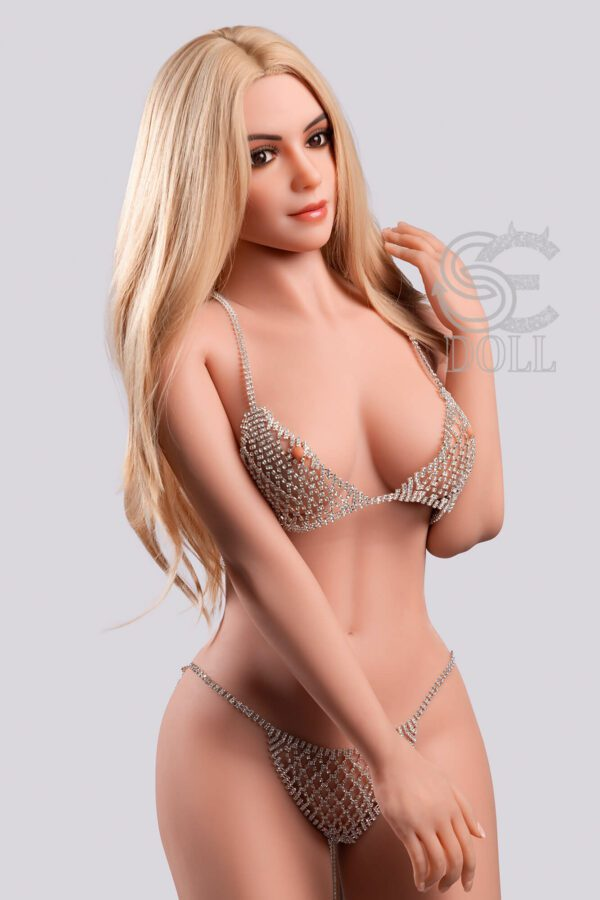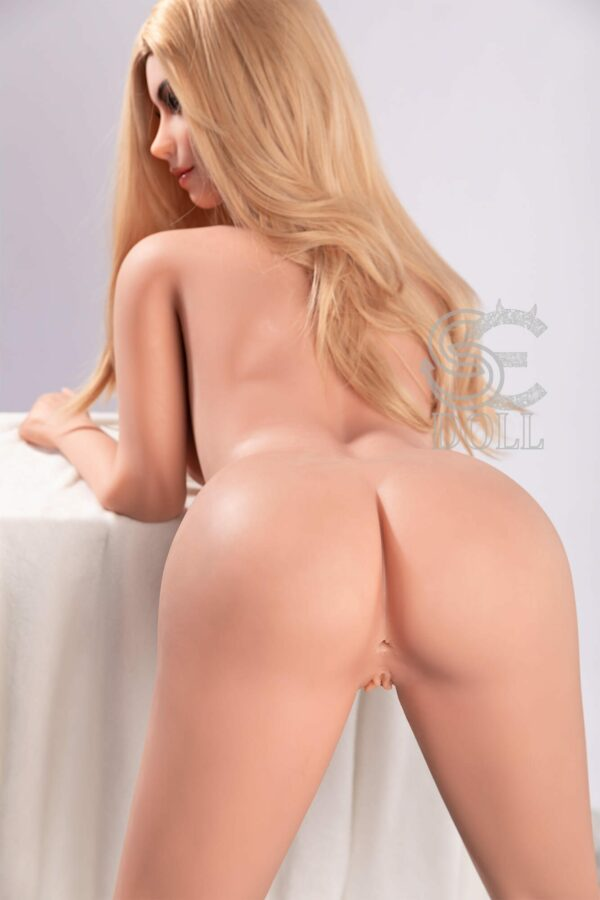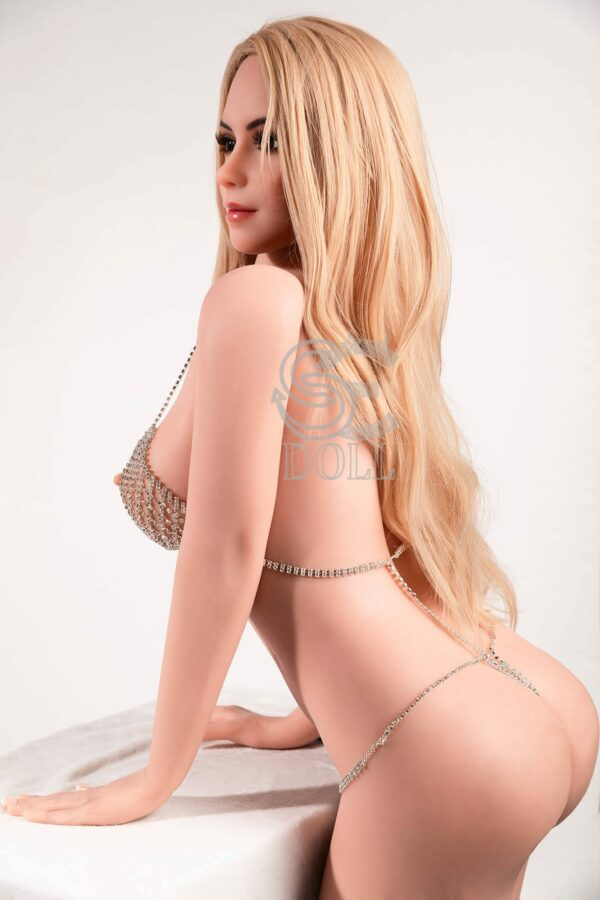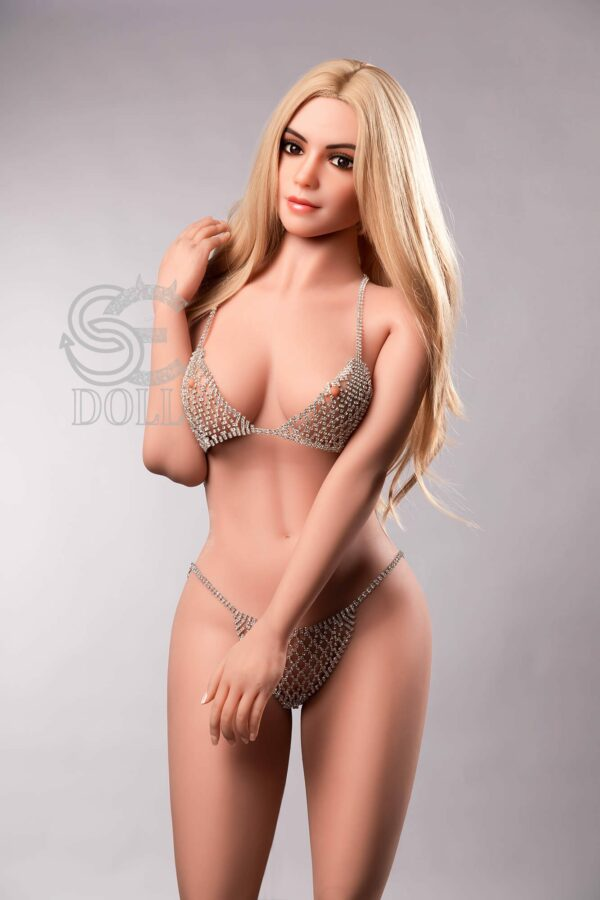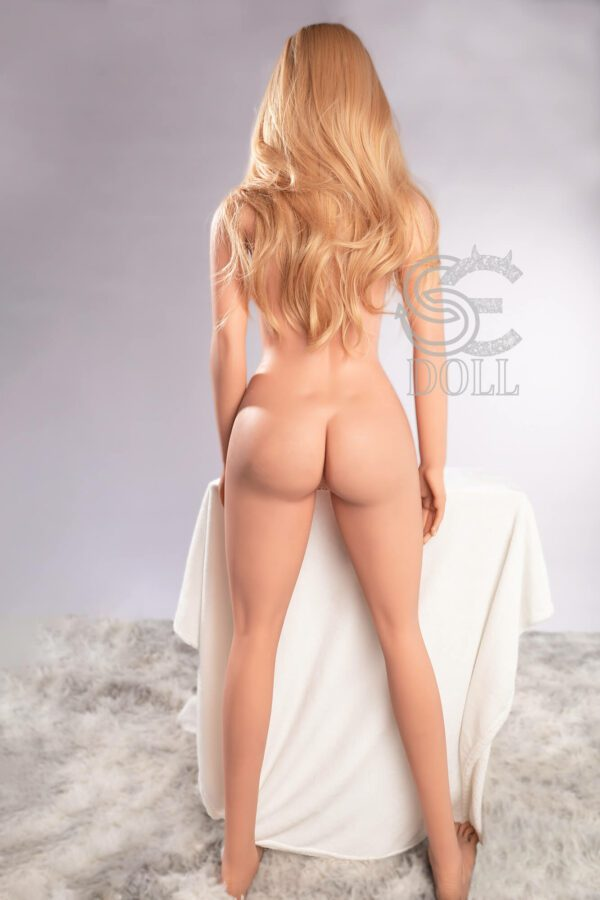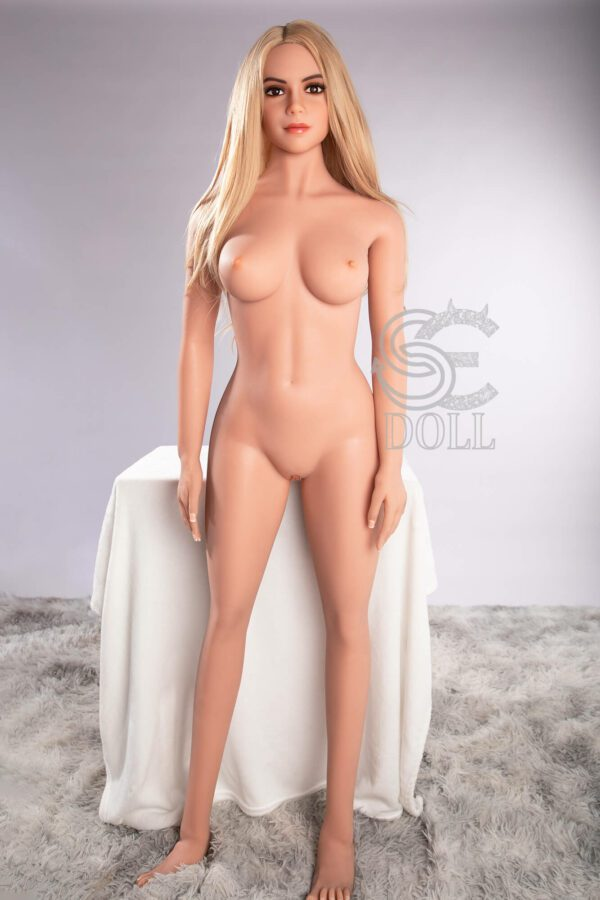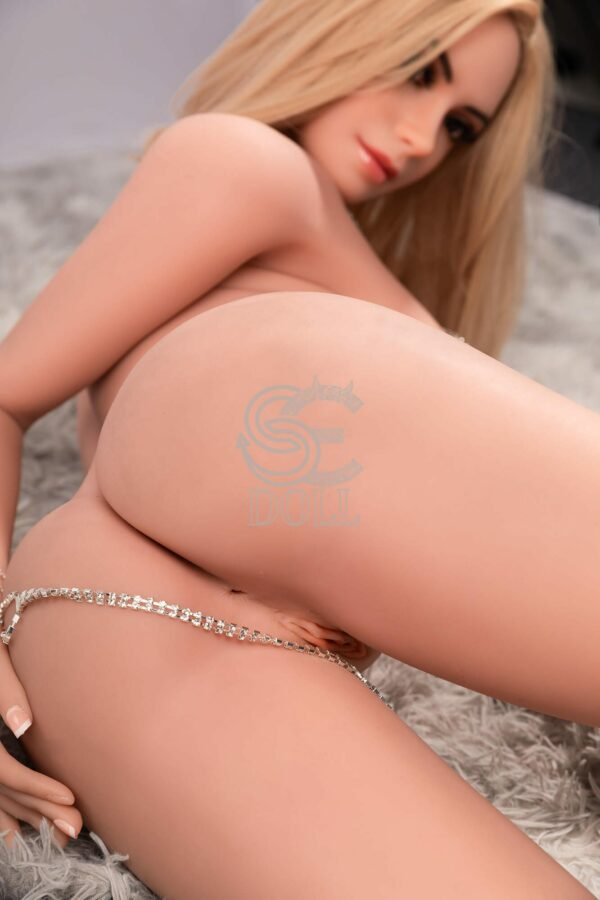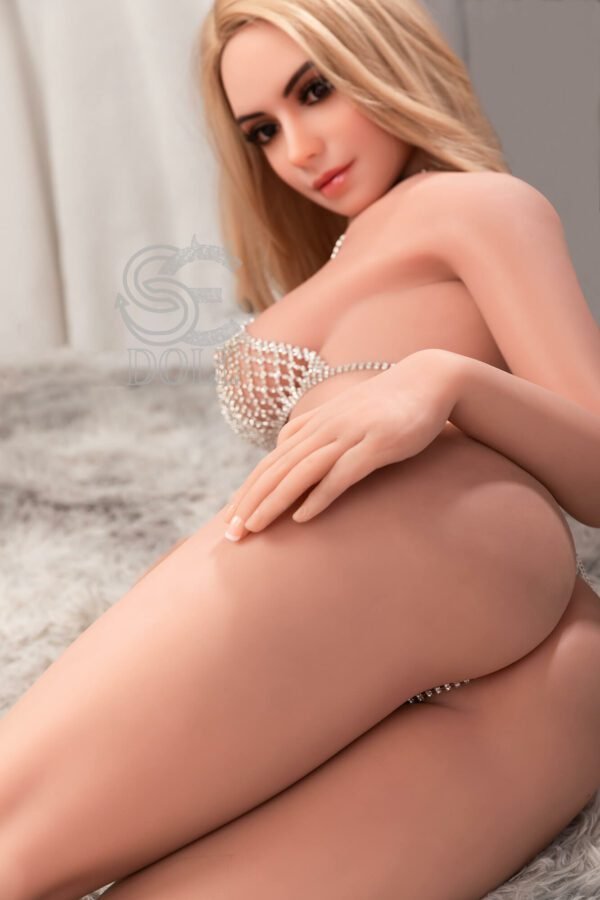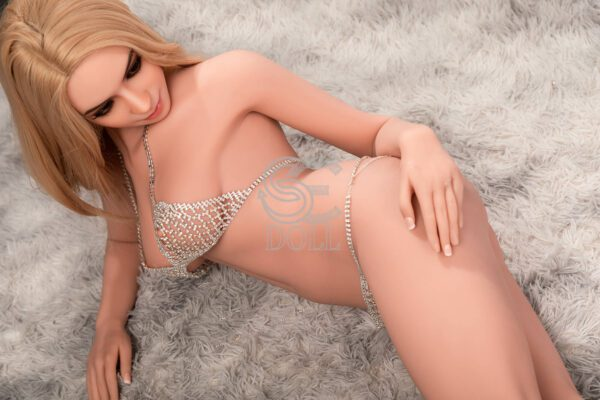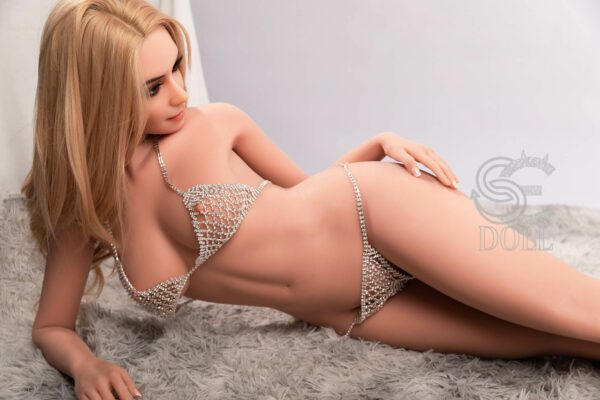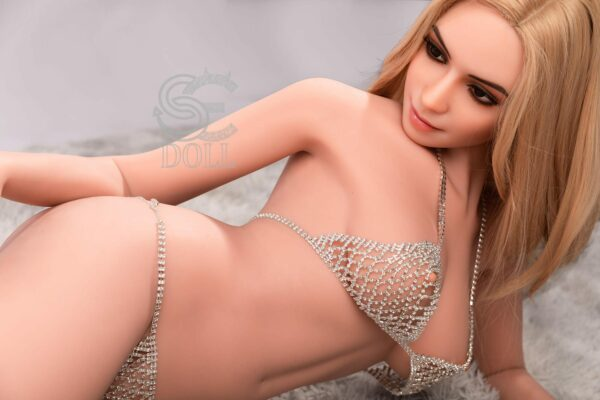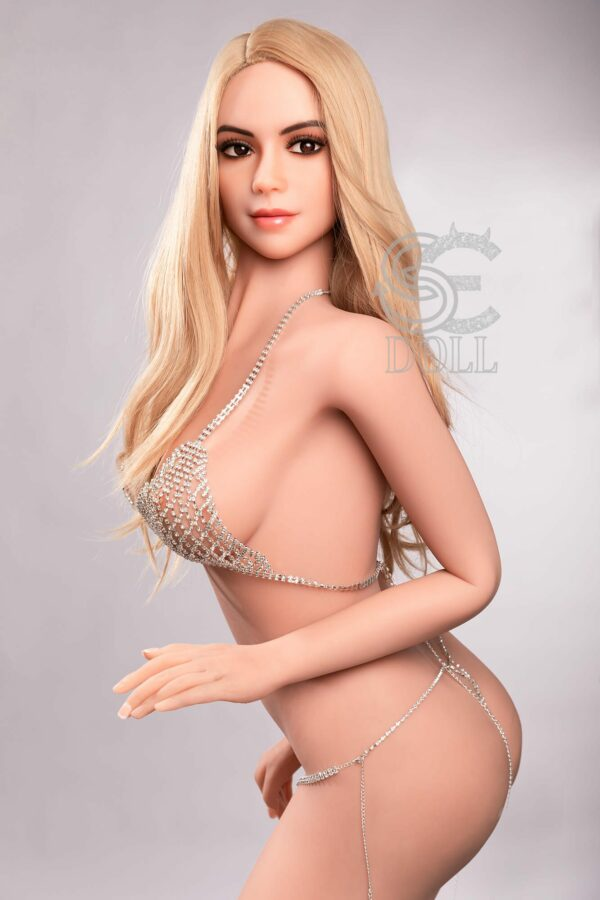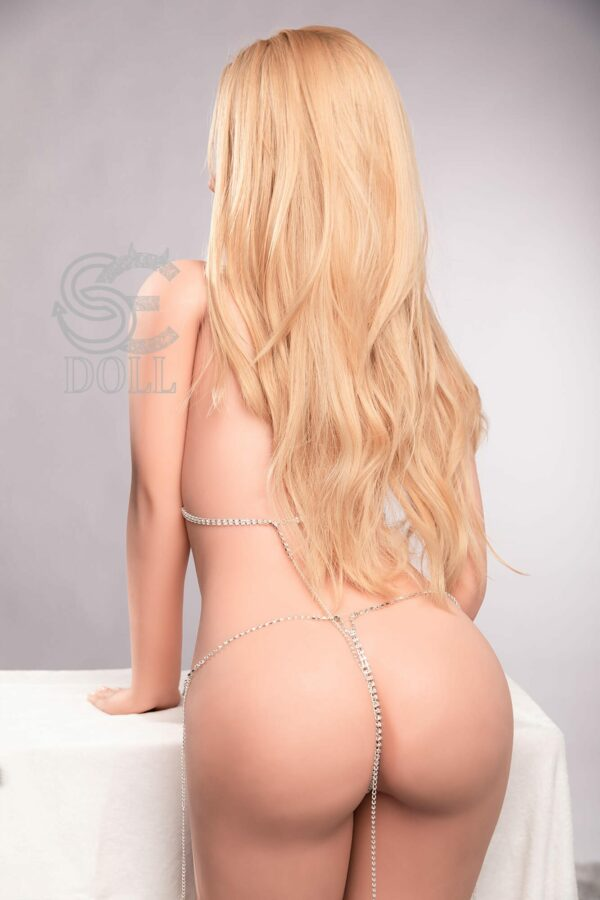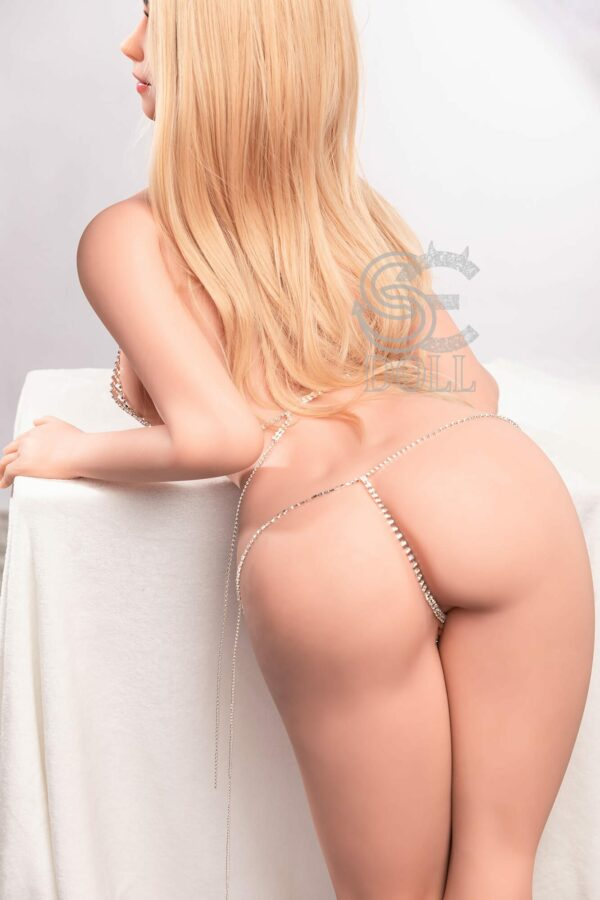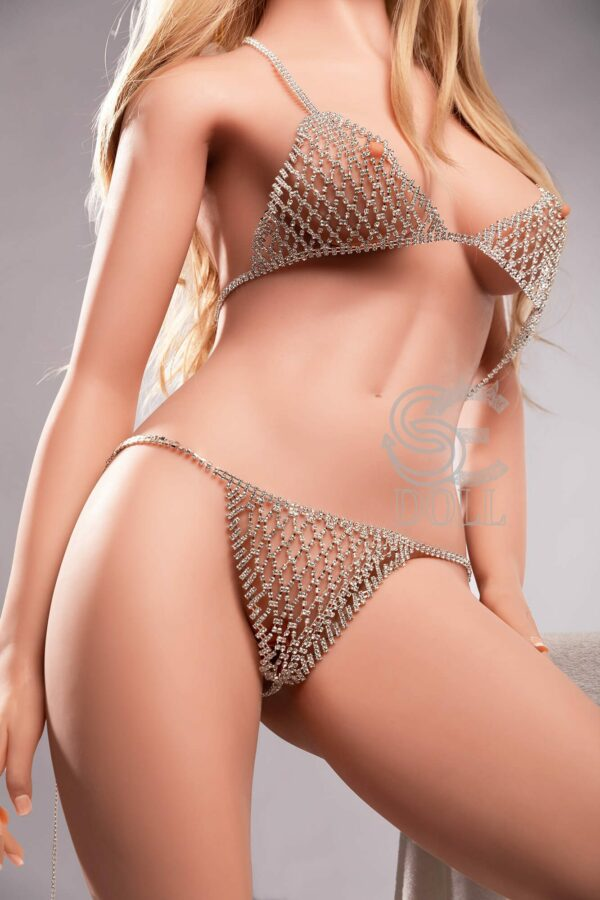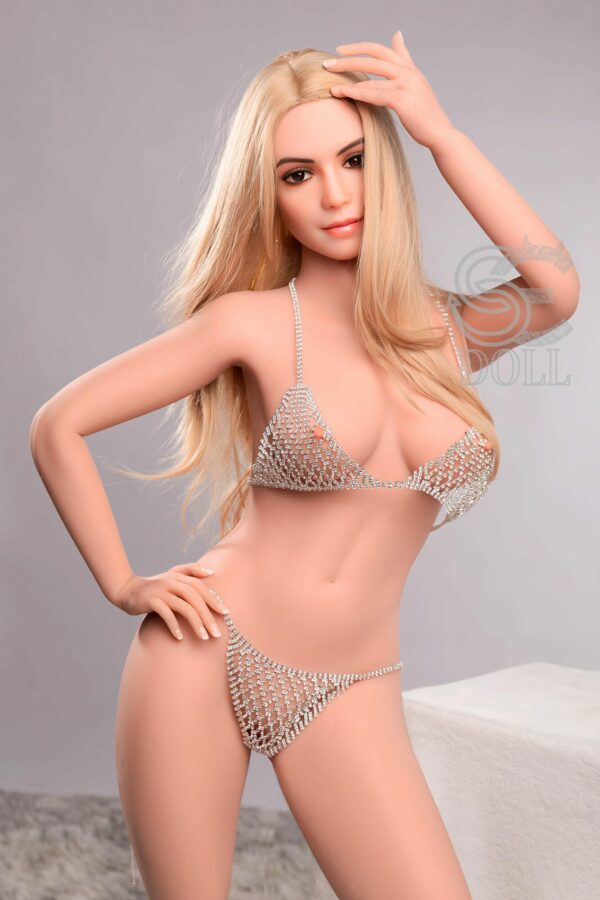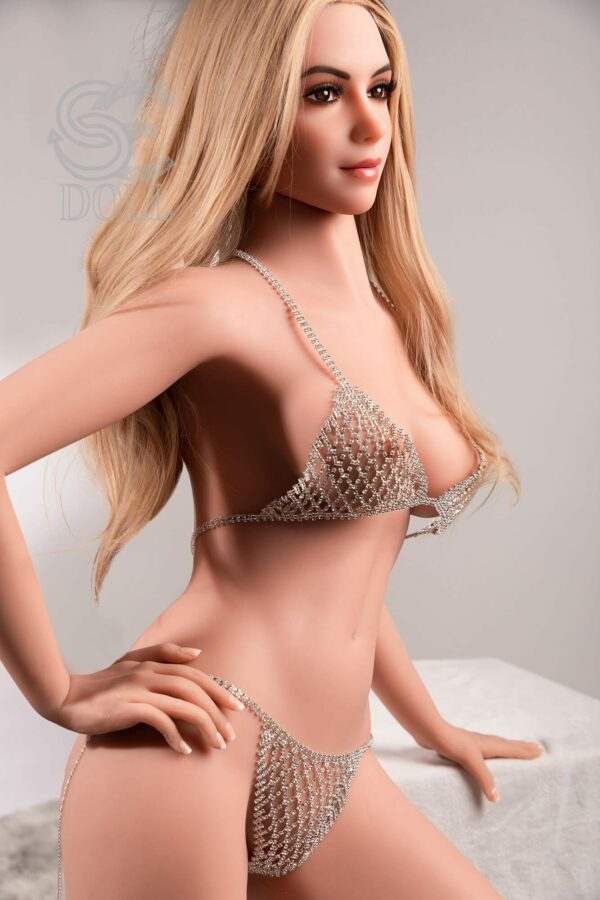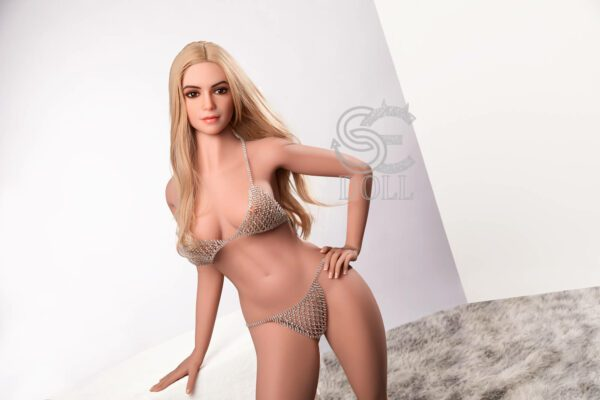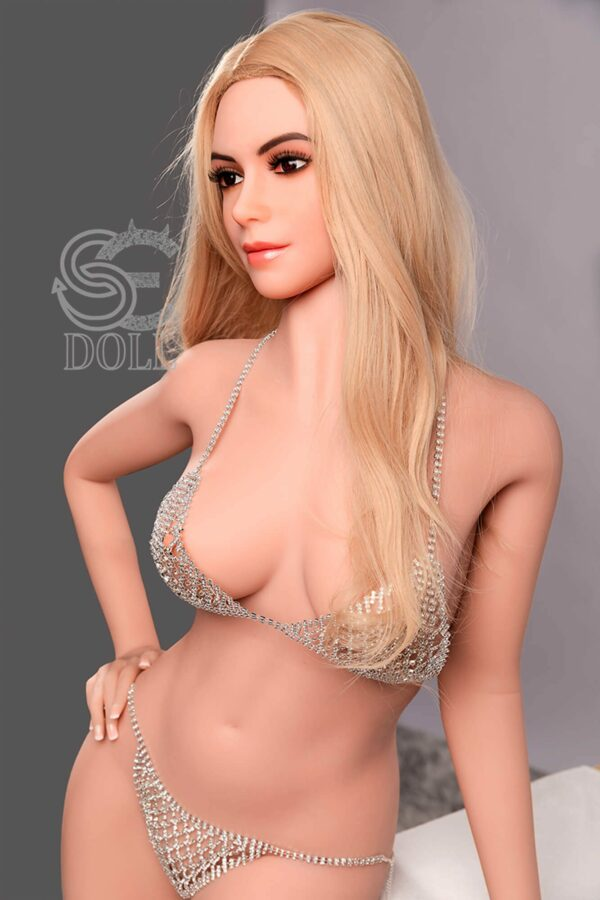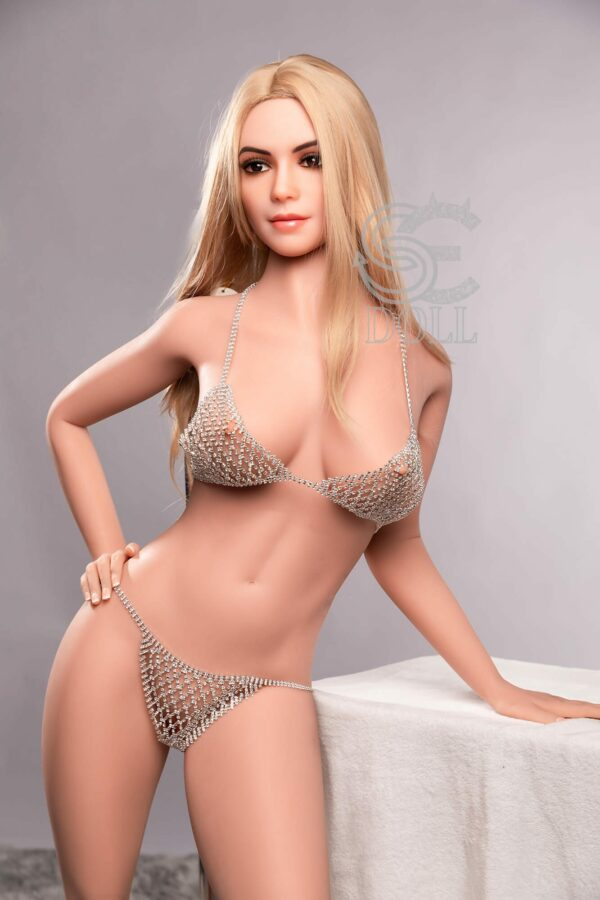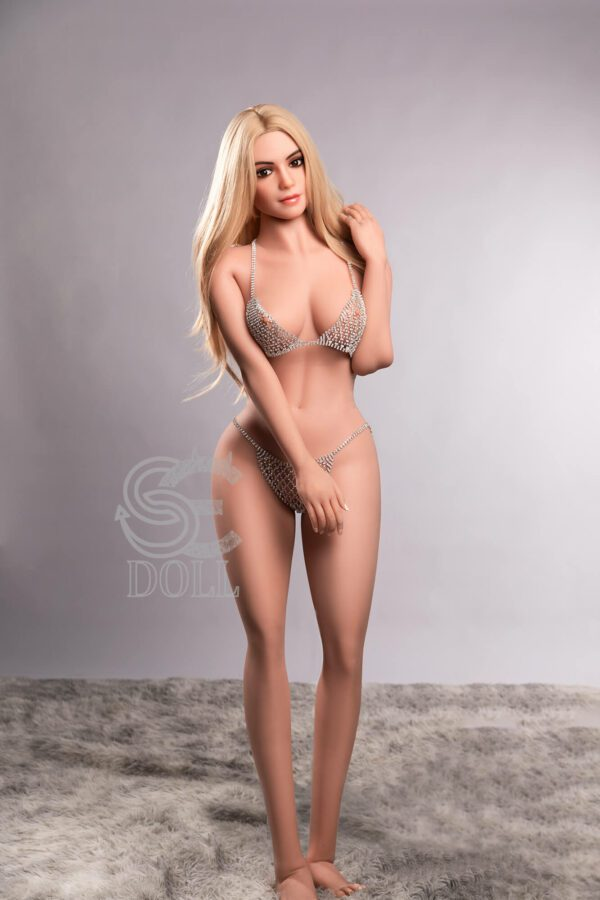# Maya – 5’4″ | 163cm SE Doll Sex Doll

SE Doll
\$2,200

Maya – 5’4″ | 163cm Life Size Sex Doll made with high quality and safety standards by SE Doll, this genuine silicone and TPE sexy real sex doll is CE certified. With various features complementing each other, this realistic sex doll is sure to please the owner.

#### Do you need financing ?

Doll Measurements Head SE#100-C Skin Color light tan Height: 161cm, 5ft3in Upper Bust: 83cm, 32.7 in Under Bust: 61cm, 24 in Bra Size: 28F Waist: 50cm, 19.7 in Hips: 89cm, 35 in Oral Depth: 15cm, 5.9 in Vagina Depth: 17cm, 6.6 in Anal Depth: 17cm, 6.6 in Foot Length: 21cm, 8.2 in Net Weight: 35kg, 77.2 lbs. Packing Size: 156*40*30cm

• \$
• \$
• \$
• \$
• \$
• \$
• \$
• \$
• \$
• \$
• \$
• \$
• \$
• \$
• \$
• \$
• \$
• \$
• \$
• \$
• \$
• \$
• \$
• \$
• \$
• \$
• \$
• \$
• \$
• \$
• \$
• \$
• \$
• \$
• \$
• \$
• \$
• \$
• \$
• \$
• \$
• \$
• \$
• \$
• \$
• \$
• \$
• \$
• \$
• \$
• \$
• \$
• \$
• \$
• \$
• \$
• \$
• \$
• \$
• \$
• \$
• \$
• \$
• \$
• \$
• \$
• \$
• \$
• \$
• \$
• \$
• \$
• \$
• \$
• \$
• \$
• \$
• \$
• \$
• \$
• \$
• \$
• \$
• \$
• \$
• \$
• \$
• \$
• \$
• \$
• \$
• \$
• \$
• \$
• \$
• \$
• \$
• \$
• \$
• \$
• \$
• \$
• \$
• \$
• \$
• \$
• \$
• \$
• \$
• \$
• \$
• \$
• \$
• \$
• \$
• \$
• \$
• \$
• \$
• \$
• \$
• \$
• \$
• \$
• \$
• \$
• \$
• \$
• \$

• \$
• \$
• \$
• \$
• \$
• \$
• \$

• \$

• \$
• \$
• \$
• \$
• \$
• \$
• \$
• \$
• \$
• \$
• \$
• \$
• \$
• \$
• \$
• \$
• \$

• \$
• \$
• \$
• \$
• \$
• \$
• \$
• \$
• \$
• \$
• \$
• \$
• \$
• \$
• \$
• \$

• \$
• \$
• \$
• \$
• \$
• \$
• \$

• \$
• 40 \$

• \$
• \$

• \$
• \$
• \$
• \$
• \$

• \$
• \$
• \$
• \$
• \$

• \$
• \$
• \$

• \$
• \$
• \$

• \$
• 100 \$

• \$
• \$

• \$
• \$
• \$
• 40 \$

• \$
• \$
• \$
• \$
• \$

• \$
• \$
• \$

• \$
• 50 \$

• \$
• \$
• \$
• \$
• \$
• \$

• \$
• 120 \$

• \$
• 120 \$

## Doll Measurements

Height: 163cm | 5ft 4 in
Bra size: 32E
Net Weight: 37KG | 81.5 lbs.
Full Bust: 88cm | 34.6 in
Under bust: 72cm | 28.3 in
Waist: 63cm | 24.8 in
Hips: 90cm | 35.4 in
Foot length: 20cm | 7.9 in
Shoulder width: 40cm | 15.7 in
Vaginal Depth: 17cm | 6.7 in
Anal Depth: 15cm | 5.9 in
Oral Depth: 15cm | 5.9 in
Packing size: 153*40*29cm